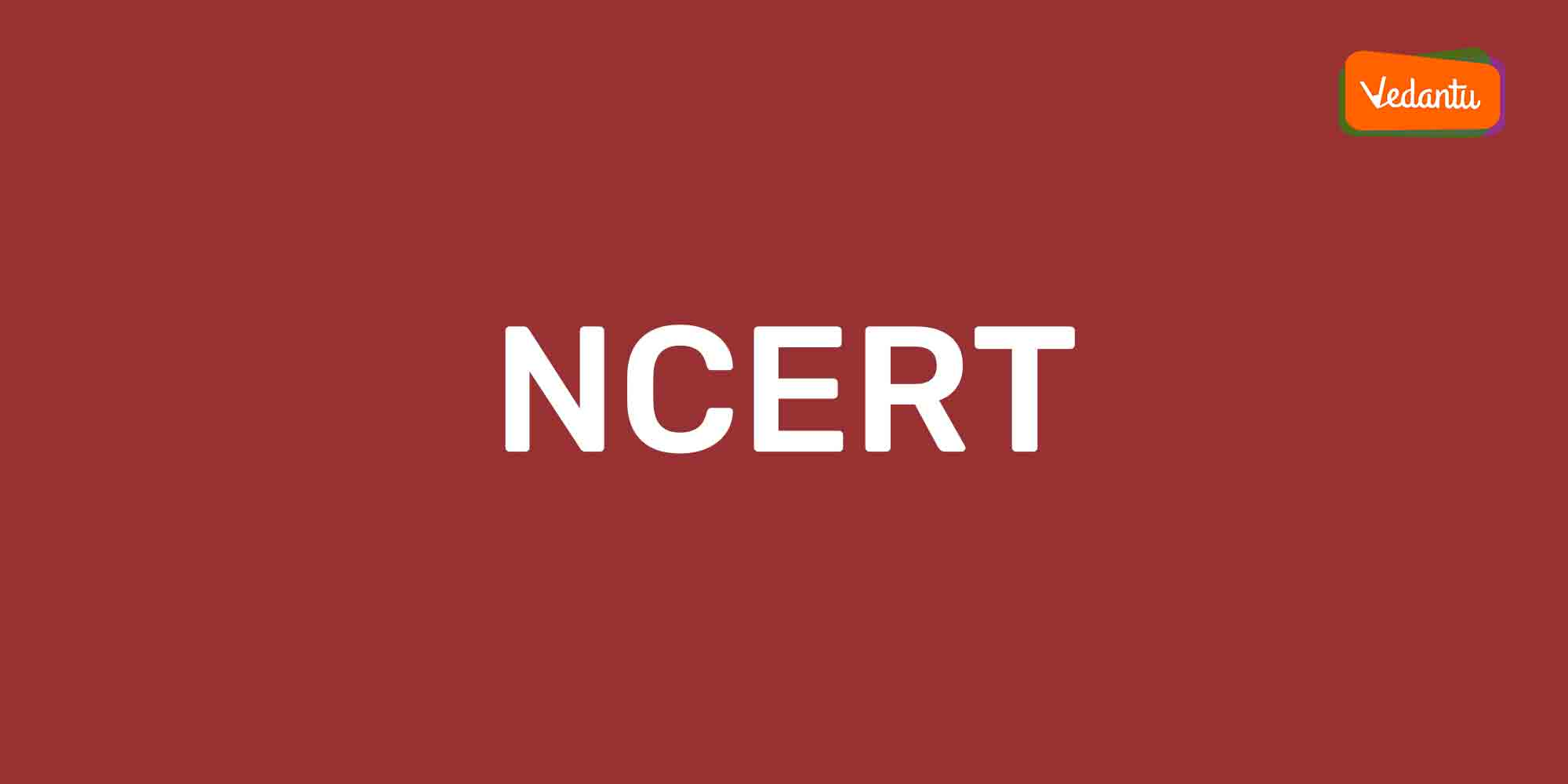# Why Toppers Use NCERT Solutions for Maths

by Asmita Kundu, May 19, 2020

Believe it or not, toppers use NCERT Solutions for maths for scoring great marks in Boards as well as in various competitive exams. They recommend you to do so as well because no matter what and how many reference books you read, unless and until your concepts are clear, you are not able to ace the tactics of solving mathematical problems. The NCERT maths solutions help you do exactly that- clear your concept of the fundamentals of maths. Now, let’s hear it from the horse’s mouth- why do toppers prefer NCERT Solutions for maths.

### NCERT Solutions for Maths

Before elucidating the reasons behind the recommendation of the toppers, first, let us tell you what NCERT Solutions for maths are and what all it covers.

NCERT Solutions for maths are generated to help you resolve all the doubt in the textbooks prescribed by NCERT. Are the NCERT Solutions for maths syllabus oriented? The NCERT solutions of maths are completely syllabus oriented and adhere to the CBSE curriculum.

### Why do Toppers use NCERT Solutions for Maths?

You will see that most of the toppers use NCERT solutions for maths and these are recommended by the experts also. When something is recommended by the persons who have gone through all the hurdles, then it must be worth following, right? The following are the reasons behind the recommendation of NCERT books by the toppers:

1. NCERT books are developed by the subject experts after rigorous research on a particular topic which helps to establish a strong foundation on all the topics.

2. You will get complete and detailed knowledge about the topic in a plain and lucid language.

3. What is special about NCERT maths solutions? NCERT maths solutions consist of all the significant topics which include a specific explanation for your better understanding.

4. There is a myth that the questions asked in the exam are directly picked from the NCERT books and NCERT solutions and take our words for it, it’s true!

5. Whether the NCERT solutions are useful for competitive exams? When toppers recommend it there should be some special element. If you are going to appear in any competitive exam in future NCERT solutions for Maths are very much useful.

6. NCERT solutions provide in-depth knowledge. That is why these are recommended by CBSE schools also.

7. Are there any solved question papers? You will find previous solved question papers and various additional problems in the NCERT solutions for maths.

8. Maths is all about practice. You need more and more questions to practise as far as the maths portion is concerned. You will find everything in a nutshell in NCERT maths solutions. What will you get? You will get numerous problems with solutions, solved previous year question papers, subject-based illustrative examples etc.

From the above discussion, the fact is clear why toppers use NCERT solutions for maths dedicatedly. Hopefully, the above article has clarified all your doubts. So, start practising NCERT textbooks and NCERT solutions to get the ultimate of maths. Remember one thing, only one word is necessary for you to get a hold of maths. That is ‘practice’, ‘practice’ and ‘practice’. If you practise the NCERT solutions rigorously one day you as a topper will recommend your fellow juniors to use NCERT solutions for maths.